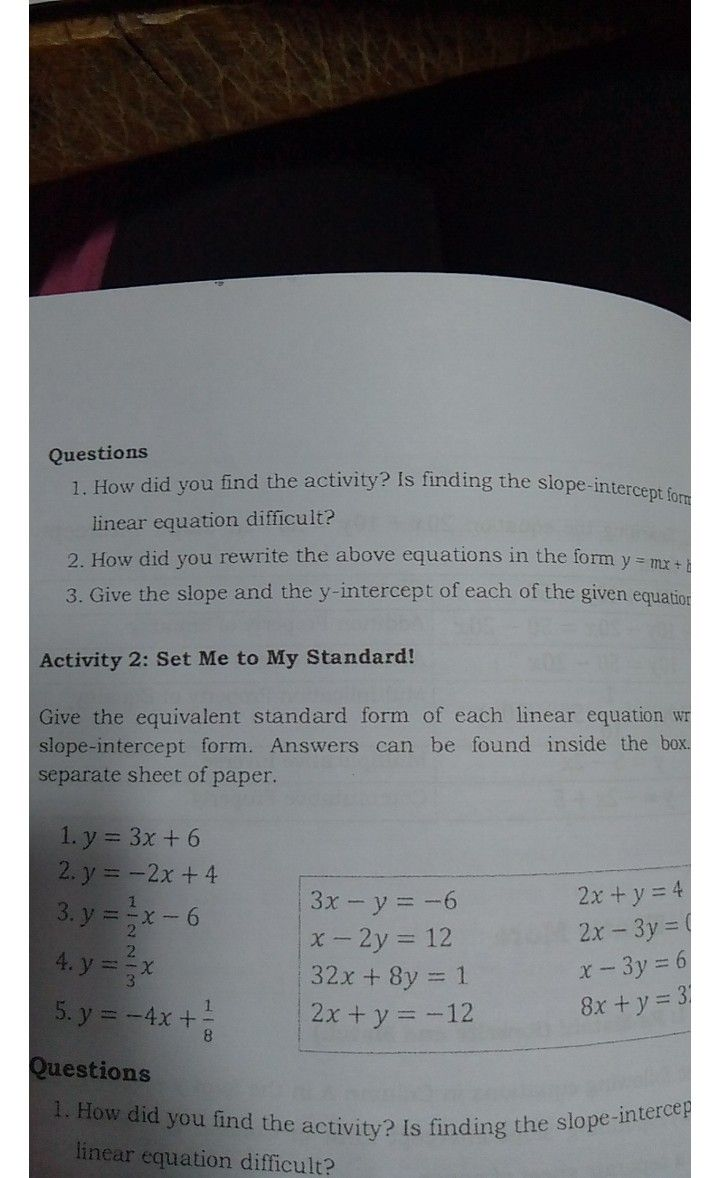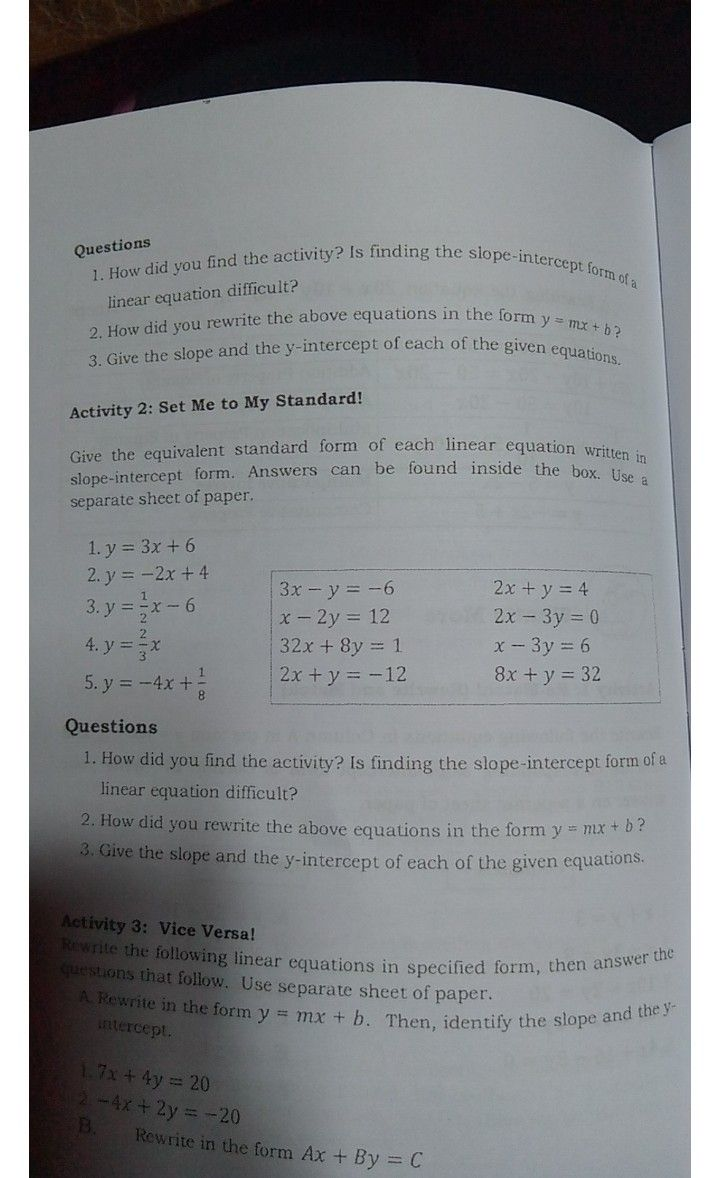Symbol
ProblemQuestions $1.H$ How did you find the activity? Is finding the $slOpe-interCep$ $\left(orn$ of a linear equation difficult? $2.$ How did you rewrite the above equations in the $\left(om$ $y=mx+b3$ $3.$ Give the slope and the $y$ $y-intercept$ of each of the given equations.
7th-9th grade
Other
Search count: 106
SolutionQanda teacher - saikumar
pls provide total question so that i can answerStudenthow about that?